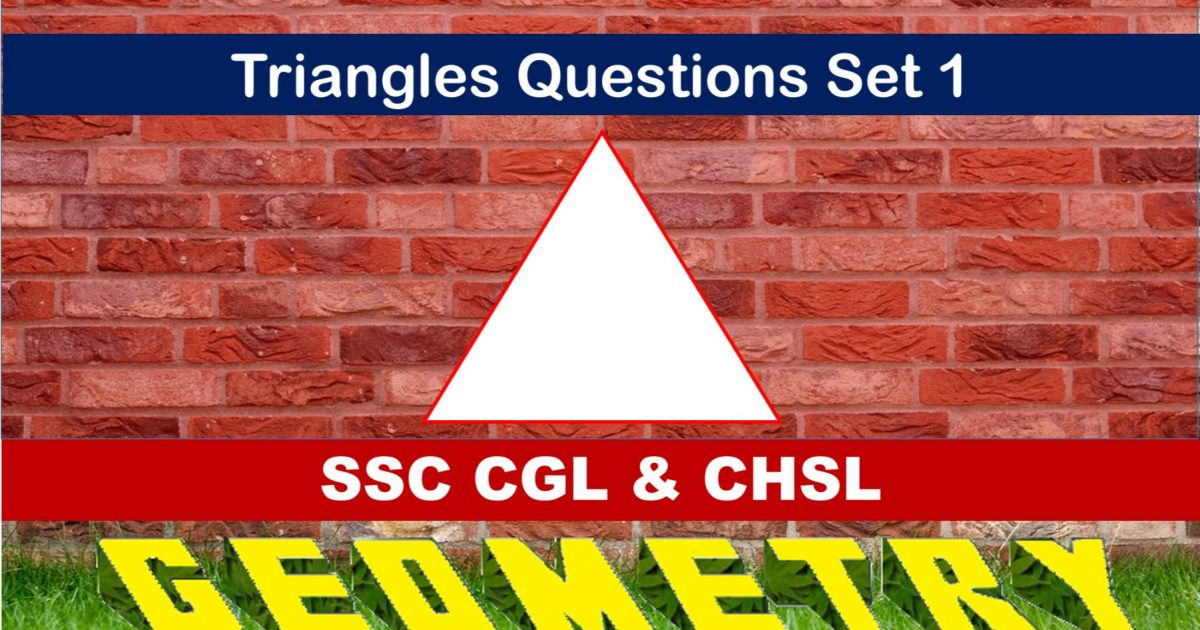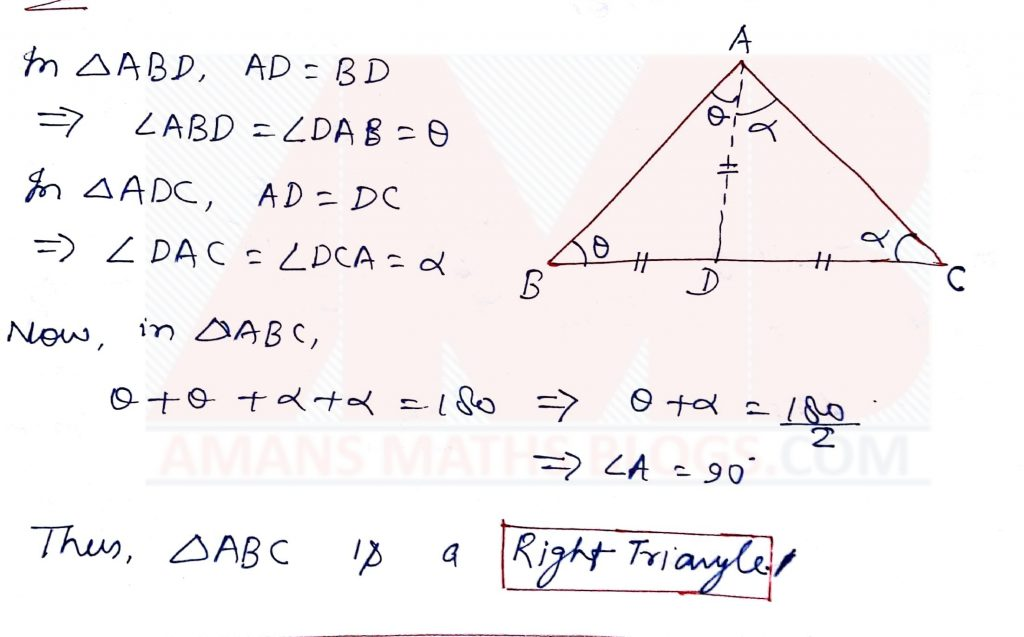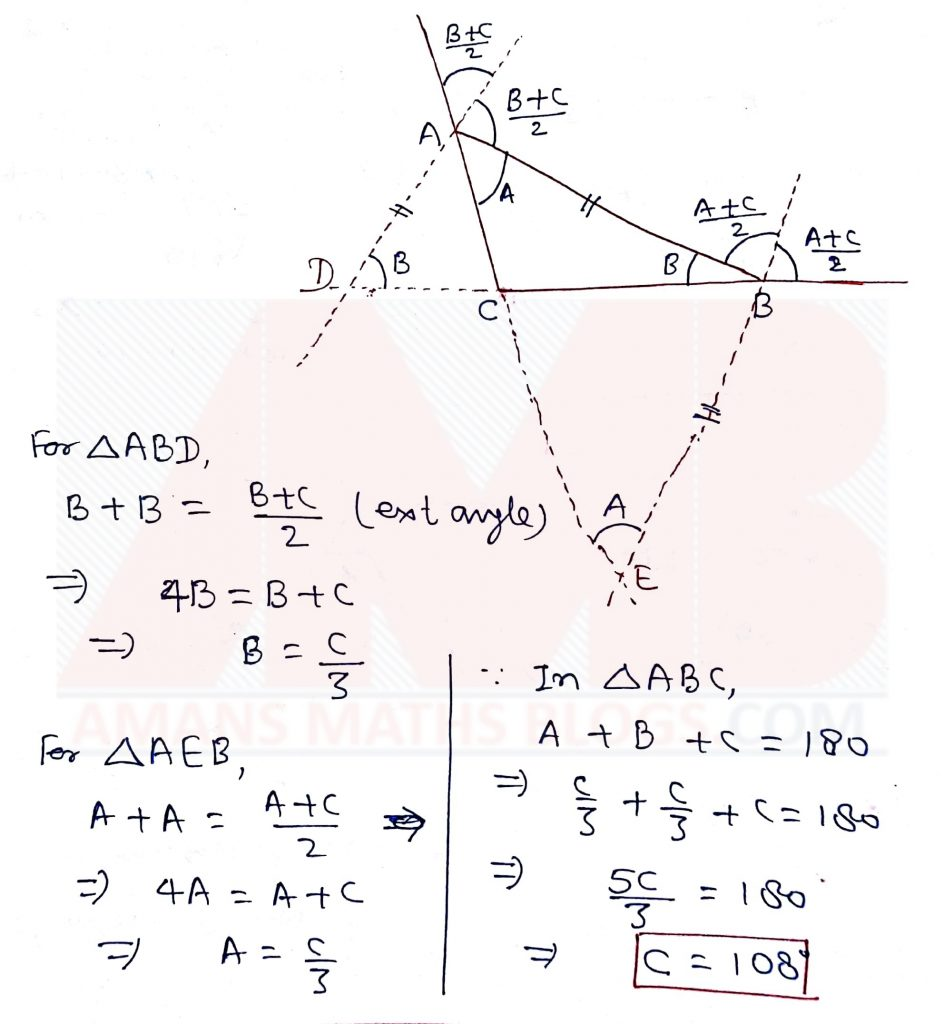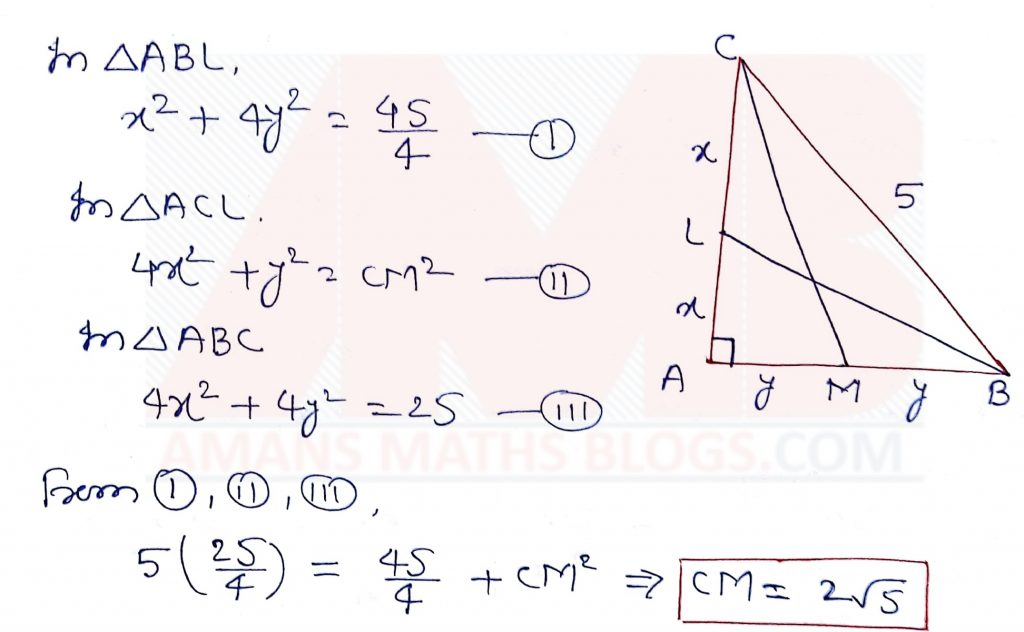Monday, September 27, 2021
Home > geometry > SSC CGL Geometry Triangle Set 1

# SSC CGL Geometry Triangle Set 1SSC CGL Geometry Triangle Set 1 : Ques No 1

∆ABC is an isosceles triangle with AB = AC and AD is the median to the base BC. If ∠ ABC = 35°, then find ∠BAD

Options:

A. 70°

B. 35°

C. 55°

D. 110°

Solution:SSC CGL Geometry Triangle Set 1 : Ques No 2

The length of 3 sides of a triangle are, 6cm, 8cm and 10cm. The length of the median to the greatest side is then,

Options:

A. 5 cm

B. 8 cm

C. 4.8 cm

D. 6 cm

Solution:SSC CGL Geometry Triangle Set 1 : Ques No 3

If I is the incenter of ∆ABC, ∠ABC = 65° and ∠ABC = 55°, then find ∠BIC.

Options:

A. 110°

B. 120°

C. 130°

D. 140°

Solution:SSC CGL Geometry Triangle Set 1 : Ques No 4

If the median drawn on the base of a triangle is half its base, the triangle will be,

Options:

A. Acute Angled Triangle

B. Obtuse Angled Triangle

C. Right Angled Triangle

D. Equilateral Triangle

Solution:SSC CGL Geometry Triangle Set 1 : Ques No 5

In a right angled triangle the product of its two sides equals half of the square of the third side which is the hypotenuse. One of the acute angles must then be

Options:

A. 15°

B. 30°

C. 45°

D. 60°

Solution:SSC CGL Geometry Triangle Set 1 : Ques No 6

AD is the median of ∆ABC and O is the centroid such that AO = 10 cm. Find the length of OD in cm

Options:

A. 7

B. 5

C. 4

D. 2

Solution:SSC CGL Geometry Triangle Set 1 : Ques No 7

A, B and C are three points on the circumference of a circle. if AB = AC = 5√2 and ∠BAC = 90°, then the value of radius is

Options:

A. 15 cm

B. 5 cm

C. 10 cm

D. 20 cm

Solution:SSC CGL Geometry Triangle Set 1 : Ques No 8

In ∆ABC, ∠C is an obtuse angle. The bisectors of exterior angles at A and B meet extended BC and AC at D and E respectively. If AB = AD = BE, then find the value of ∠ACB

Options:

A. 120 degrees

B. 108 degrees

C. 105 degrees

D. 135 degrees

Solution:SSC CGL Geometry Triangle Set 1 : Ques No 9

How many triangles can be formed by taking any three from the four line segments of length 2 cm, 3 cm, 5 cm and 6 cm?

Options:

A. 1

B. 2

C. 3

D. 4

Solution:SSC CGL Geometry Triangle Set 1 : Ques No 10

In right triangle ABC, BL and Cm are two medians with right angle at ∠A and BC = 5 cm. If BL = (3√5)/2, then the length of CM is

Options:

A. 2√5 cm

B. 10√2 cm

C. 5√2 cm

D. 4√5 cm

Solution:SSC CGL Geometry Triangle Set 1 : Ques No 11

The ratio of the sides of a triangle is 5 : 12 : 13. If the difference between the largest side and the smallest side of the triangle is 1.6. Then, the area of the triangle is

Options:

A. 2.4

B. 1.2

C. 1.8

D. 2.6

SSC CGL Geometry Triangle Set 1 : Ques No 12

If the side BC of a triangle ABC is extended up to D such that CD = AC and  angle BAD = 109° and angle ACB = 72°, then the value of angle ABC is

Options:

A. 35 degree

B. 60 degree

C. 40 degree

D. 45 degree